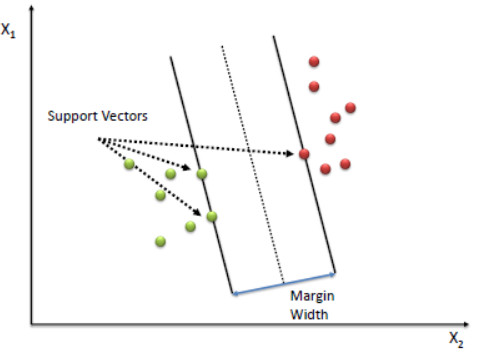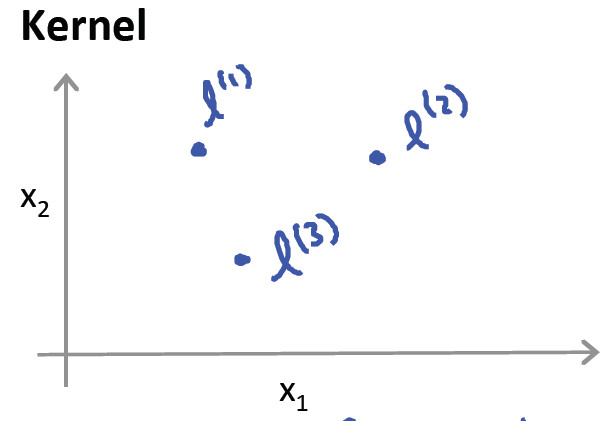# What is support vector machine/SVM

Last updated on：2 years ago

SVM is usually mentioned in Machine Learning. But sometimes I still get confused that how it relates to ML.

# Definition

In machine learning, support vector machines/SVMs are supervised learning models with associated learning algorithms that analyse data for classification and regression analysis.

It follows the idea, Input vectors are non-linearly mapped to a very high-dimension feature space

Also, the neural network is a learning model of machine learning. Different learning models with a different cost function, characteristics, application.

Support vector machine is a large margin classifier.

A Support Vector Machine (SVM) performs classification by finding the hyperplane that maximizes the margin between the two classes. The vectors (cases) that define the hyperplane are the support vectors.# SVM hypothesis

$$\min_\theta C \sum^{m}_{i=1} [y^{(i)} cost_1 (\theta^T x^{(i)}) + (1 - y^{(i)}) cost_0( \theta^T x^{(i)})] + \frac{1}{2} \sum^{n}_{i=1} \theta_j^2$$

## Need to specify

• Choice of parameter C
• Choice of kernel (similarity function)

For C, remember: if C is larger, $\theta$ or $\omega$ is larger, then the model is going to overfit

# Kernels

Adapt SVM to develop complex nonlinear classifier

$$f_i = \text{similarity} (x, l^{(i)}) = exp ( - \frac{|| x - l^{(i)}|| ^2}{2 \sigma ^2})$$

Superscript is still the level of layer.## Kernel types

Linear kernel
$$\theta_0 + \theta_1 x_1 + … + \theta_n x_n \ge 0$$

Polynomial kernel

$$k(x,l) = (x^T l)^2, (x^T l)^3, (x^T l+1)^2, (x^T l + \text{constant})^{\text{degree}}$$
More esoteric

string k, chi-square k, histogram intersection k

# Logistic regression vs SVM

• If n is larger than m, use logistic regression or SVM without a kernel
With so many features, linear functions can fit very complicated non-linear function
• If n is small, m is intermediate
Use SVM with Gaussian kernel
• If n is small, m is large
Create/add more features, then use logistic regression or SVM without a kernel

Neural network likely to work well for most of these settings, but maybe slower to train.

# SVM in deep learning

Replace softmax by SVM.

Note that prediction using SVMs is exactly the same as using a softmax.

The only difference between softmax and multiclass SVMs is in their objectives parametrized by all of the weight matrices W. Soft- max layer minimizes cross-entropy or maximizes the log-likelihood, while SVMs simply try to find the maximum margin between data points of different classes.

## Multiclass problem

The dominant approach for doing so is to reduce the single multiclass problem into multiple binary classification problems.

Each two classes combination has a identical decision boundary.

# Reference

 Andrew NG, Machine learning

 Tang, Y., 2013. Deep learning using linear support vector machines. arXiv preprint arXiv:1306.0239.